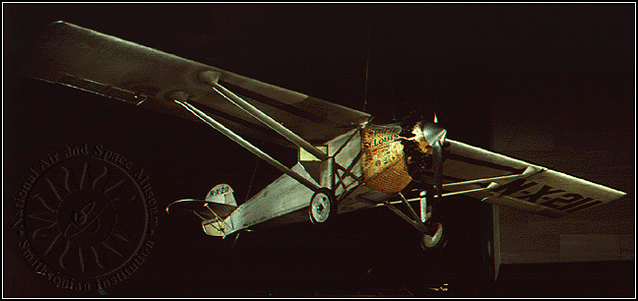+ Text Only Site
+ Non-Flash Version
+ Contact GlennLift/Velocity Relationship Problems If so instructed by your teacher, print out a worksheet page for these problems. Open the slide called Velocity Effects (with text) and read the explanation on how lift varies with the square of the velocity. At the bottom of the Mass and Velocity Effects slide is a problem that supplies weight and velocity data. Let's work through that problem and discover the effect a large change in weight has upon velocity. The problem gives us a 15,000 pound airplane with 5,000 pounds of fuel traveling at 250 mph. The lift must equal a total weight of 20,000 pounds, which is equal to the velocity squared multiplied by some constant (assuming constant air density) . Therefore, we have L = k V2. Solving for k, k = L/ V2 , and substituting in our data, k = 20,000 pounds/ (250 mph)2 = 0.32 pounds-hours2/mile2. Now we wish to know the velocity the pilot should maintain to hold the same altitude after he has burned half of his fuel. Our weight (and necessarily lift) becomes 17,500 pounds, which is then equal to 0.32 V2. Solving for V, V = (17,500/0.32)1/2 = 234 mph.You may have been wondering about the photo of the "Spirit of St. Louis" above. We will now use our lift/velocity relationship information on an imaginary flight in the Spirit of St. Louis where we again maintain altitude. Data needed to continue is found at "Ryan, Spirit of St. Louis." (http://en.wikipedia.org/wiki/Spirit_of_St._Louis)   Find "k" as above using the Spirit of St. Louis maximum velocity and the takeoff weight less 20 kg (an arbitrary amount of fuel to attain maximum velocity). Once you have determined k, write an equation for velocity as a function of lift (as in our example). V= __________.   Using a function grapher, graph this equation (default window settings are acceptable). Do you recognize the graph? It is a ______________.   Now key in the following RANGE window values: xmin=1000, xmax=2500, xscl=100, ymin=120, ymax=220, yscl=10, and graph the equation. Because the domain and range are limited to real Spirit of St. Louis values, the curvature of the parabola segment is limited.   Return to the equation and solve it for lift. L= ________________. Graph the equation. Then exchange the x and y values in the RANGE window; that is, key in: xmin=120, xmax=220, xscl=10, ymin=1000, ymax=2500, yscl=100, and graph. The parabola segment now opens upwards, instead of to the side. Note: You may wish to try this for other aircraft

Related Pages:
Standards
Worksheet
Lesson Index
Aerodynamics Index+ Inspector General Hotline + Equal Employment Opportunity Data Posted Pursuant to the No Fear Act + Budgets, Strategic Plans and Accountability Reports + Freedom of Information Act + The President's Management Agenda + NASA Privacy Statement, Disclaimer, and Accessibility CertificationEditor: Tom Benson NASA Official: Tom Benson Last Updated: Thu, Jun 12 04:46:37 PM EDT 2014 + Contact Glenn